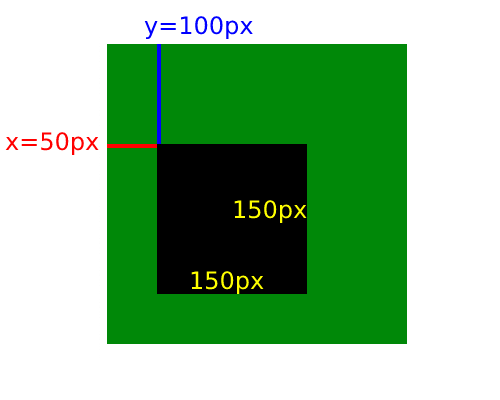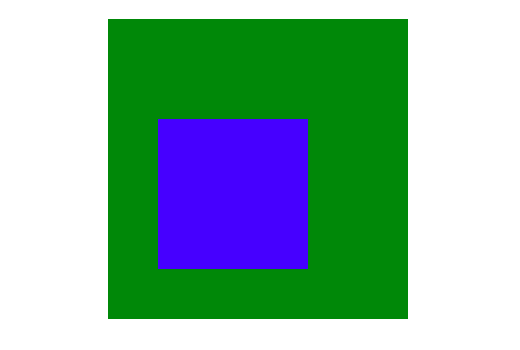# The fillRect() JavaScript method explained

Learn how to draw a rectangle with JavaScript using the fillRect() method.

Posted on March 25, 2021

The `fillRect()` method is a method from Canvas API that allows you to draw a colored rectangle on top of the HTML `<canvas>` element. To use the `fillRect()` method, you need to first grab the `Context` object of a `<canvas>` element that has to be present in your HTML file.

The `fillRect()` method requires the following 4 `number` parameters:

• The `x` parameter to specify the x-axis coordinate of the rectangle’s starting point
• The `y` parameter represents the y-axis coordinate of the rectangle’s starting point
• The `width` parameter to specify the rectangle’s width. Positive values will cause the width expanding to the right, negative values to the left
• The `height` parameter to specify the rectangle’s height. Positive values will cause the height to expand up, negative values to expand down.

The parameters will be counted in pixels. The full syntax is as follows:

``````Context.fillRect(x, y, width, height);
``````

Sometimes, you’ll see the `Context` object shortened as `ctx` in JavaScript code. We’ll use the `ctx` for the rest of this tutorial. Let’s see how the `fillRect()` method actually works.

Suppose you have the following HTML `<body>` tag in your project:

``````<body>
<canvas
id="emptyCanvas"
width="300"
height="300"
style="background-color: green"
></canvas>
</body>
``````

The `style="background-color: green"` is added so that you can see the size of the `<canvas>` element on your HTML file. To grab the context of the `<canvas>` element, you need to use the `getContext()` method from the `<canvas>` itself as follows:

``````let canvas = document.getElementById("emptyCanvas");
let ctx = canvas.getContext('2d');
ctx.fillRect(50, 100, 150, 150);
``````

The `getContext('2d')` method returns the two-dimensional representation of the rendered context, so you can create a 2D drawing on it. Next, you just need to call `ctx.fillRect()` and pass the desired size for your rectangle.

The code example above will draw a rectangle starting at `x` 50 pixels and `y` 100 pixels. The `width` and `height` of the rectangle will be 150 pixels:A black rectangle drawn by fillRect() method

As you can see, the drawn rectangle is black because that’s the default color of the `fillStyle` attribute:

``````let canvas = document.getElementById("emptyCanvas");
let ctx = canvas.getContext('2d');
console.log(ctx.fillStyle); // #000000
``````

To change the color of the rectangle, you can assign a different color from the CSS `color` value. For example, you can pass `"blue"` as the `fillStyle` value to draw a blue rectangle:

``````let canvas = document.getElementById("emptyCanvas");
let ctx = canvas.getContext('2d');
ctx.fillStyle = "blue";
ctx.fillRect(50, 100, 150, 150);
``````

The output of the code above will be as follows:A blue rectangle drawn by fillRect() method

And that’s how you use the `fillRect()` method to draw a rectangle with JavaScript and HTML `<canvas>` element.

Related articles:

### Grab the free JavaScript book today 👍

Learn the building blocks of JavaScript programming language like data types, functions, objects, arrays and classes.

Use the knowledge from the book to build a small but solid program.